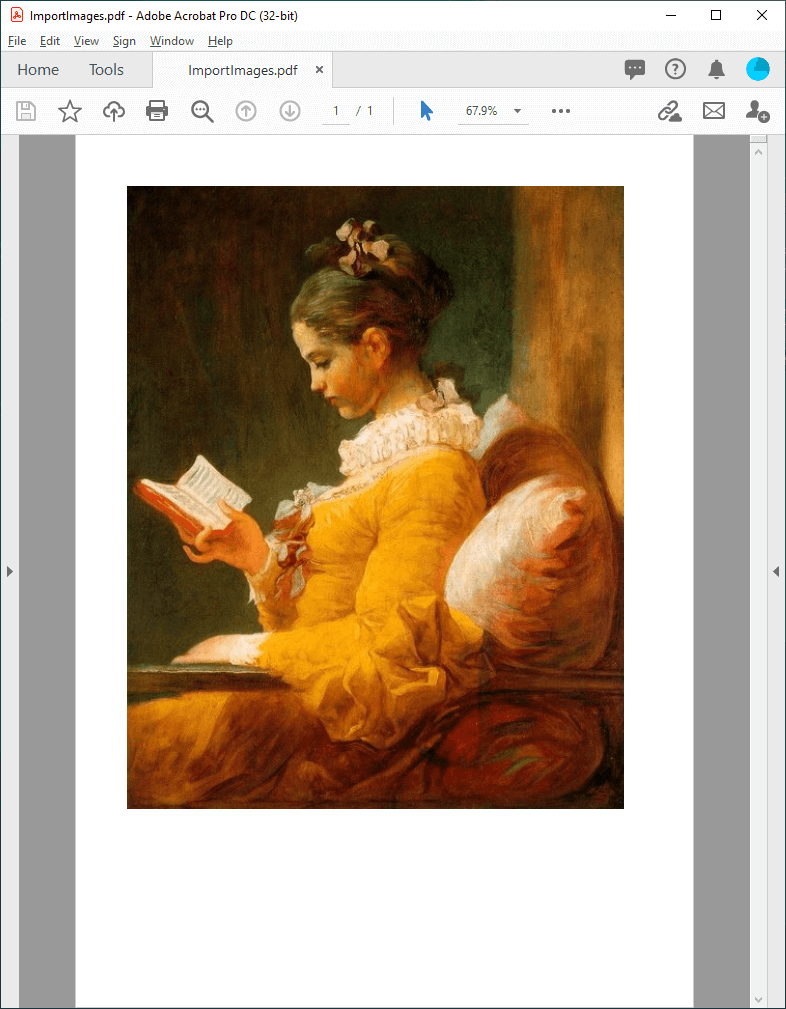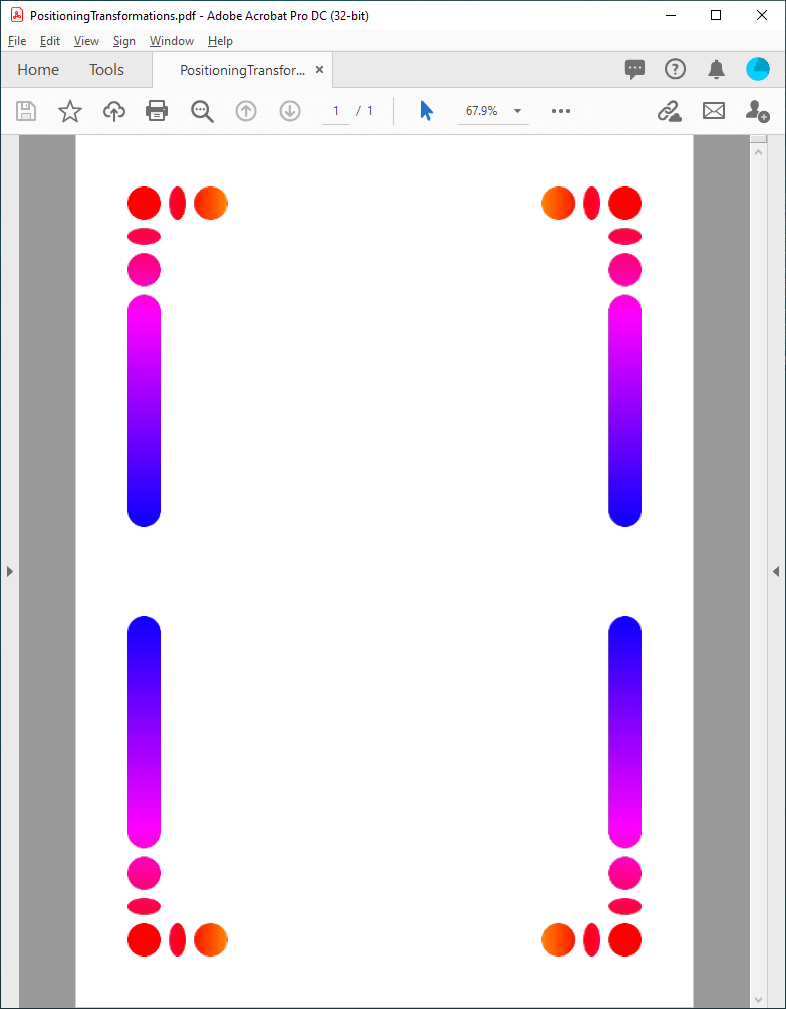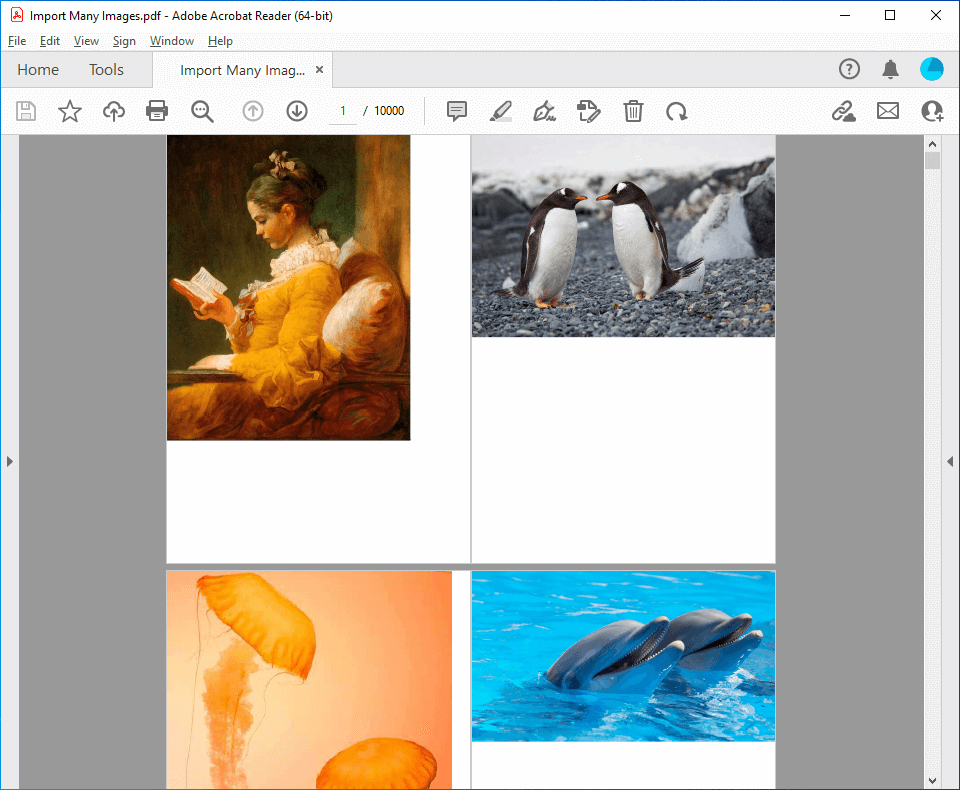# Export and import images to PDF file in C# and VB.NET

## Export images

GemBox.Pdf supports exporting images from the PDF file in JPEG, BMP, PNG or TIFF image formats.

The following example shows how you can export single image from the PDF file.

``````using System.Linq;
using GemBox.Pdf;
using GemBox.Pdf.Content;

class Program
{
static void Main()
{
// If using the Professional version, put your serial key below.

{
// Iterate through PDF pages.
foreach (var page in document.Pages)
{
// Get all image content elements on the page.
var imageElements = page.Content.Elements.All().OfType<PdfImageContent>().ToList();

// Export the first image element to an image file.
if (imageElements.Count > 0)
{
imageElements.Save("Export Images.%OutputFileType%");
break;
}
}
}
}
}``````
``````Imports System.Linq
Imports GemBox.Pdf
Imports GemBox.Pdf.Content

Module Program

Sub Main()

' If using the Professional version, put your serial key below.

' Iterate through PDF pages.
For Each page In document.Pages

' Get all image content elements on the page.
Dim imageElements = page.Content.Elements.All().OfType(Of PdfImageContent)().ToList()

' Export the first image element to an image file.
If imageElements.Count > 0 Then
imageElements(0).Save("Export Images.%OutputFileType%")
Exit For
End If

Next

End Using

End Sub
End Module``````

## Export image locations

The following example shows how you can retrieve the location and size of images in the PDF file.

``````using System;
using GemBox.Pdf;
using GemBox.Pdf.Content;

class Program
{
static void Main()
{
// If using the Professional version, put your serial key below.

{
// Iterate through all PDF pages and through each page's content elements,
// and retrieve only the image content elements.
for (int index = 0; index < document.Pages.Count; index++)
{
var page = document.Pages[index];
var contentEnumerator = page.Content.Elements.All(page.Transform).GetEnumerator();
while (contentEnumerator.MoveNext())
{
if (contentEnumerator.Current.ElementType == PdfContentElementType.Image)
{
var imageElement = (PdfImageContent)contentEnumerator.Current;
Console.Write(\$"Image on page {index + 1} | ");

var bounds = imageElement.Bounds;
contentEnumerator.Transform.Transform(ref bounds);
Console.Write(\$"from ({bounds.Left:#},{bounds.Bottom:#}) to ({bounds.Right:#},{bounds.Top:#}) | ");

var image = imageElement.Image;
Console.WriteLine(\$"size {image.Size.Width}x{image.Size.Height}");
}
}
}
}
}
}``````
``````Imports System
Imports GemBox.Pdf
Imports GemBox.Pdf.Content

Module Program

Sub Main()

' If using the Professional version, put your serial key below.

' Iterate through all PDF pages and through each page's content elements,
' and retrieve only the image content elements.
For index As Integer = 0 To document.Pages.Count - 1
Dim page = document.Pages(index)
Dim contentEnumerator = page.Content.Elements.All(page.Transform).GetEnumerator()

While contentEnumerator.MoveNext()
If contentEnumerator.Current.ElementType = PdfContentElementType.Image Then
Dim imageElement = CType(contentEnumerator.Current, PdfImageContent)
Console.Write(\$"Image on page {index + 1} | ")

Dim bounds = imageElement.Bounds
contentEnumerator.Transform.Transform(bounds)
Console.Write(\$"from ({bounds.Left},{bounds.Bottom}) to ({bounds.Right},{bounds.Top}) | ")

Dim image = imageElement.Image
Console.WriteLine(\$"size {image.Size.Width}x{image.Size.Height}")
End If
End While
Next

End Using

End Sub
End Module``````

## Import images

GemBox.Pdf supports importing images in a PDF file from your C# or VB.NET application.

The following example shows how you can easily create a PDF document and import an image to its first page.Screenshot of PDF file that contains imported image created with GemBox.Pdf library
``````using GemBox.Pdf;
using GemBox.Pdf.Content;

class Program
{
static void Main()
{
// If using the Professional version, put your serial key below.

using (var document = new PdfDocument())
{

// Load the image from a file.

// Set the location of the bottom-left corner of the image.
// We want the top-left corner of the image to be at location (50, 50)
// from the top-left corner of the page.
// NOTE: In PDF, location (0, 0) is at the bottom-left corner of the page
// and the positive y axis extends vertically upward.
double x = 50, y = page.CropBox.Top - 50 - image.Size.Height;

// Draw the image to the page.
page.Content.DrawImage(image, new PdfPoint(x, y));

document.Save("Import Images.%OutputFileType%");
}
}
}``````
``````Imports GemBox.Pdf
Imports GemBox.Pdf.Content

Module Program

Sub Main()

' If using the Professional version, put your serial key below.

Using document = New PdfDocument()

' Load the image from a file.

' Set the location of the bottom-left corner of the image.
' We want the top-left corner of the image to be at location (50, 50)
' from the top-left corner of the page.
' NOTE: In PDF, location (0, 0) is at the bottom-left corner of the page
' and the positive y axis extends vertically upward.
Dim x As Double = 50, y As Double = page.CropBox.Top - 50 - image.Size.Height

' Draw the image to the page.
page.Content.DrawImage(image, New PdfPoint(x, y))

document.Save("Import Images.%OutputFileType%")
End Using

End Sub
End Module``````

## Image positioning and transformations

The following example shows how to position and apply various transformations, such as rotation or scaling, to an image drawn on a PDF page.Screenshot of PDF file that contains positioned and transformed image created with GemBox.Pdf library
``````using GemBox.Pdf;
using GemBox.Pdf.Content;

class Program
{
static void Main()
{
// If using the Professional version, put your serial key below.

using (var document = new PdfDocument())
{

// Load the image from a file.

double margin = 50;

// Set the location of the first image in the top-left corner of the page (with a specified margin).
double x = margin;
double y = page.CropBox.Top - margin - image.Size.Height;

// Draw the first image.
page.Content.DrawImage(image, new PdfPoint(x, y));

// Set the location of the second image in the top-right corner of the page (with the same margin).
x = page.CropBox.Right - margin - image.Size.Width;
y = page.CropBox.Top - margin - image.Size.Height;

// Initialize the transformation.
var transform = PdfMatrix.Identity;
// Use the translate operation to position the image.
transform.Translate(x, y);
// Use the scale operation to resize the image.
// NOTE: The unit square of user space, bounded by user coordinates (0, 0) and (1, 1),
// corresponds to the boundary of the image in the image space.
transform.Scale(image.Size.Width, image.Size.Height);
// Use the scale operation to flip the image horizontally.
transform.Scale(-1, 1, 0.5, 0);

// Draw the second image.
page.Content.DrawImage(image, transform);

// Set the location of the third image in the bottom-left corner of the page (with the same margin).
x = margin;
y = margin;

// Initialize the transformation.
transform = PdfMatrix.Identity;
// Use the translate operation to position the image.
transform.Translate(x, y);
// Use the scale operation to resize the image.
transform.Scale(image.Size.Width, image.Size.Height);
// Use the scale operation to flip the image vertically.
transform.Scale(1, -1, 0, 0.5);

// Draw the third image.
page.Content.DrawImage(image, transform);

// Set the location of the fourth image in the bottom-right corner of the page (with the same margin).
x = page.CropBox.Right - margin - image.Size.Width;
y = margin;

// Initialize the transformation.
transform = PdfMatrix.Identity;
// Use the translate operation to position the image.
transform.Translate(x, y);
// Use the scale operation to resize the image.
transform.Scale(image.Size.Width, image.Size.Height);
// Use the scale operation to flip the image horizontally and vertically.
transform.Scale(-1, -1, 0.5, 0.5);

// Draw the fourth image.
page.Content.DrawImage(image, transform);

document.Save("Positioning and Transformations.%OutputFileType%");
}
}
}
``````
``````Imports GemBox.Pdf
Imports GemBox.Pdf.Content

Module Program

Sub Main()

' If using the Professional version, put your serial key below.

Using document = New PdfDocument()

' Load the image from a file.

Dim margin As Double = 50

' Set the location of the first image in the top-left corner of the page (with a specified margin).
Dim x As Double = margin
Dim y As Double = page.CropBox.Top - margin - image.Size.Height

' Draw the first image.
page.Content.DrawImage(image, New PdfPoint(x, y))

' Set the location of the second image in the top-right corner of the page (with the same margin).
x = page.CropBox.Right - margin - image.Size.Width
y = page.CropBox.Top - margin - image.Size.Height

' Initialize the transformation.
Dim transform = PdfMatrix.Identity
' Use the translate operation to position the image.
transform.Translate(x, y)
' Use the scale operation to resize the image.
' NOTE: The unit square of user space, bounded by user coordinates (0, 0) and (1, 1),
' corresponds to the boundary of the image in the image space.
transform.Scale(image.Size.Width, image.Size.Height)
' Use the scale operation to flip the image horizontally.
transform.Scale(-1, 1, 0.5, 0)

' Draw the second image.
page.Content.DrawImage(image, transform)

' Set the location of the third image in the bottom-left corner of the page (with the same margin).
x = margin
y = margin

' Initialize the transformation.
transform = PdfMatrix.Identity
' Use the translate operation to position the image.
transform.Translate(x, y)
' Use the scale operation to resize the image.
transform.Scale(image.Size.Width, image.Size.Height)
' Use the scale operation to flip the image vertically.
transform.Scale(1, -1, 0, 0.5)

' Draw the third image.
page.Content.DrawImage(image, transform)

' Set the location of the fourth image in the bottom-right corner of the page (with the same margin).
x = page.CropBox.Right - margin - image.Size.Width
y = margin

' Initialize the transformation.
transform = PdfMatrix.Identity
' Use the translate operation to position the image.
transform.Translate(x, y)
' Use the scale operation to resize the image.
transform.Scale(image.Size.Width, image.Size.Height)
' Use the scale operation to flip the image horizontally And vertically.
transform.Scale(-1, -1, 0.5, 0.5)

' Draw the fourth image.
page.Content.DrawImage(image, transform)

document.Save("Positioning and Transformations.%OutputFileType%")
End Using
End Sub
End Module
``````

The above example shows how to add multiple instances of the same image on a single page. To add multiple instances of the same image on multiple pages, see the Watermarks example.

## Import a large number of images

GemBox.Pdf enables you to work efficiently with a large number of PDF images. With the lazy loading and incremental update features, you can utilize less memory when processing the documents.

The `PdfImage` objects are loaded when requested for the first time and with `PdfDocument.Unload` method, you can clear previously loaded images.

Also, with the `PdfDocument.Save` method you can save the changes made to the current document in the same location from where the document was loaded or last saved.

The following example shows how you can import many images into a single PDF file by utilizing the `PdfDocument`'s lazy loading and incremental update.Screenshot of large number of images imported into one PDF file
``````using System;
using System.IO;
using GemBox.Pdf;
using GemBox.Pdf.Content;

class Program
{
static void Main()
{
// If using the Professional version, put your serial key below.

var imageFiles = Directory.EnumerateFiles("Images");

int imageCounter = 0;
int chunkSize = 1000;

using (var document = new PdfDocument())
{
// Create output PDF file that will have large number of images imported into it.
document.Save("Import Many Images.pdf");

foreach (var imageFile in imageFiles)
{

double ratioX = page.Size.Width / image.Width;
double ratioY = page.Size.Height / image.Height;
double ratio = Math.Min(ratioX, ratioY);

var imageSize = ratio < 1 ?
new PdfSize(image.Width * ratio, image.Height * ratio) :
new PdfSize(image.Width, image.Height);
var imagePosition = new PdfPoint(0, page.Size.Height - imageSize.Height);
page.Content.DrawImage(image, imagePosition, imageSize);

++imageCounter;
if (imageCounter % chunkSize == 0)
{
// Save the new images that were added after the document was last saved.
document.Save();

// Clear previously parsed images and thus free memory necessary for merging additional pages.
}
}

// Save the last chunk of imported images.
document.Save();
}
}
}``````
``````Imports System
Imports System.IO
Imports GemBox.Pdf
Imports GemBox.Pdf.Content

Module Program

Sub Main()

' If using the Professional version, put your serial key below.

Dim imageFiles = Directory.EnumerateFiles("Images")

Dim imageCounter As Integer = 0
Dim chunkSize As Integer = 1000

Using document = New PdfDocument()

' Create output PDF file that will have large number of images imported into it.
document.Save("Import Many Images.pdf")

For Each imageFile In imageFiles

Dim ratioX As Double = page.Size.Width / image.Width
Dim ratioY As Double = page.Size.Height / image.Height
Dim ratio As Double = Math.Min(ratioX, ratioY)

Dim imageSize = If(ratio < 1,
New PdfSize(image.Width * ratio, image.Height * ratio),
New PdfSize(image.Width, image.Height))
Dim imagePosition = New PdfPoint(0, page.Size.Height - imageSize.Height)
page.Content.DrawImage(image, imagePosition, imageSize)

imageCounter += 1
If imageCounter Mod chunkSize = 0 Then

' Save the new images that were added after the document was last saved.
document.Save()

' Clear previously parsed images and thus free memory necessary for merging additional pages.